# Python Program To Reverse A Number

In this tutorial, we will see Python programs to reverse a number. For example, if the input is 95401, then the output should be 10459. We can apply multiple logics to reverse a number in Python. Following are the methods we are going to explore in this Python tutorial:

1. While loop
2. For loop
3. Recursion
4. String slicing
5. Reversed method

## Python Program To Reverse A Number Using While Loop

Code:

```Num = 95401
ReverseNumber = 0

while Num != 0:
digit = Num % 10
ReverseNumber = ReverseNumber * 10 + digit
Num //= 10

print("Reversed Number: " + str(ReverseNumber))```

Output: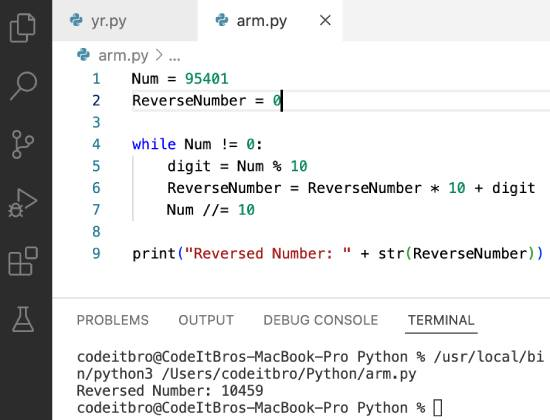## Python Program to Reverse a Number Using For Loop

Code:

```def reverse_for_loop(per):
emptyString = ''
for i in per:
emptyString = i + emptyString
return emptyString
num = '95401'
if __name__ == "__main__":
print('Reversing the given number using for loop =', reverse_for_loop(num))```

Output: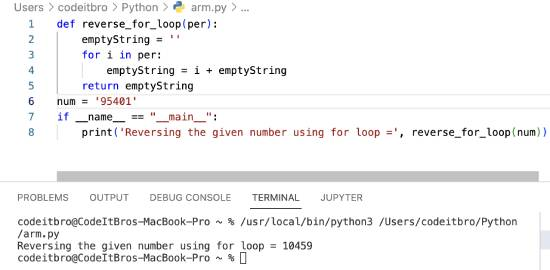## Python Program to Reverse a Number Using Recursion

Code:

```def reverse(n, r):
if n==0:
return r
else:
return reverse(n//10, r*10 + n%10)

num = int(input("Enter number: "))

reverseNum = reverse(num,0)

print("Reverse of %d is %d" %(num, reverseNum))```

Output: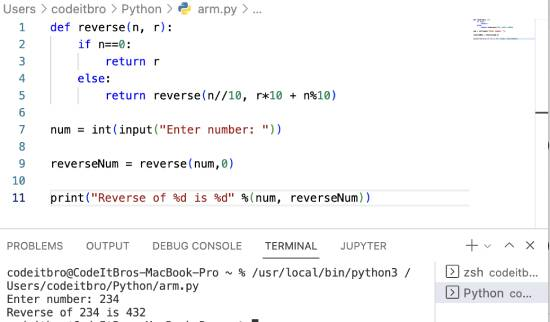## Python Program to Reverse a Number Using String Slicing

Code:

```num = 95401
print(str(num)[::-1])```

Output: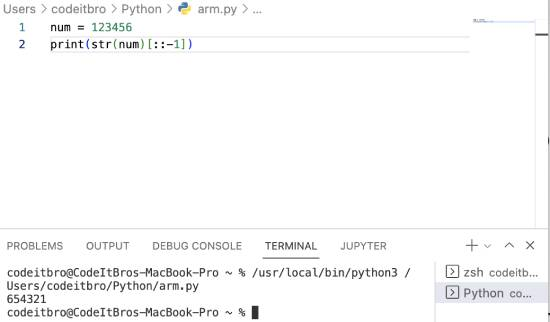## Python Program to Reverse a Number Using Reversed Method

Code:

```def reverse(string):
string = "".join(reversed(string))
return string
num = "95401"
print (num)
print ("Reverse of the given number is : ",end="")
print (reverse(num))```

Output: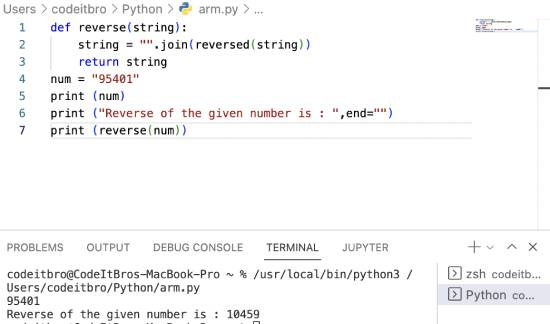Also ReadWhat Does The Python Percent Sign Mean?

## Python Program To Reverse A Number Using Function

Code:

```def ReverseNum():
Num = 95401
ReverseNumber = 0
while Num != 0:
digit = Num % 10
ReverseNumber = ReverseNumber * 10 + digit
Num //= 10
print("Reversed Number: " + str(ReverseNumber))

ReverseNum()```

Output: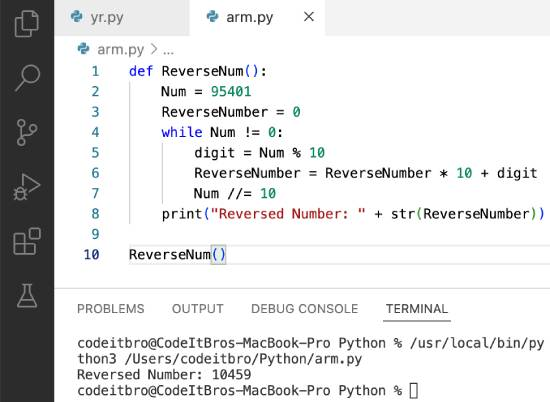Also ReadHow to Create an Empty Array In Python

### Summary

In this Python tutorial, we explored how to reverse a number using various methods such as while loop, for loop, string slicing, recursion, and custom function.

Other related tutorials:

Scroll to Top